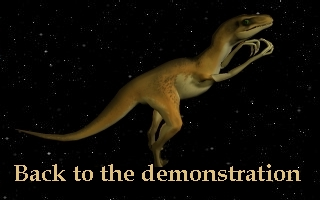The gravitational  constant G

G is what physicians call a "fundamental constante ", a value which doesn't change through time and through space.
Because of this value we can know the strength of the gravitation, the force existing between two bodies attracting each other.
If one body is the earth and the other one an object, or a beeing, placed on the earth the name of this force is...the weight!

The equation giving the value of the force is:   F =       -Gmm'
r 2

Where  G= 6,672*10-11 N.m2.kg-2

m is the mass of the first body , m' the mass of the second body. m may be the Earth's mass.
r is the distance between the center of gravity of the two objects.
N means newtons - a unit of force -, m meterss, kg  kilograms.

When G stops being a constante...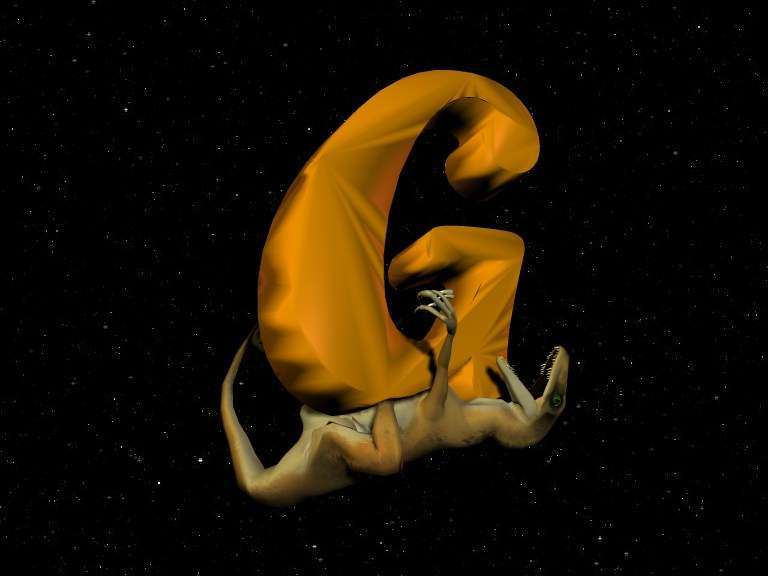Difference between weight and mass:

The mass of a body doesn't depend on where is that body. An elephant or a dinosaur will always have the same mass, never mind if he is on the Earth, on the Moon or anywhere in space.  But the weight of the animal depend on where is this animal. The weight of an elephant is not the same on the Moon or on the Earth.
The weight depends on the value of G too!
The weight of a elephant on the Earth changes if you change the value of G.
In our hypothesis, the value of G changed 65 millions years ago, the dinosaurs' weight - like other animals weights - increased!

The size of the change of G.

We need more studies to know exactly the old value of G.

But we can try to make an estimation.

If the elephant is the heaviest animal able to live, we must suppose the diplodocus had nearly the same weight than an elephant, nearly 10 tons; so the gravity during the dinosaurs' age should have been the third of todays gravity.
65 millions years ago, G, the gravity, increased threefold!

G and the "missing mass" of the universe!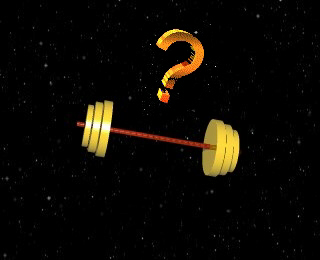For many years, astronoms are looking for the "missing mass" of the Universe. The meaning of this quest is simple: According to today's knowledge of Universe and gravity, Universe should...falls in pieces. We can't explain, for instance, why galaxies are stable.  If me make the hypothesis tha G is not a constante, we can understand why the universe is stable without looking for a "missing mass" that nobody has never found!

G and the superstrings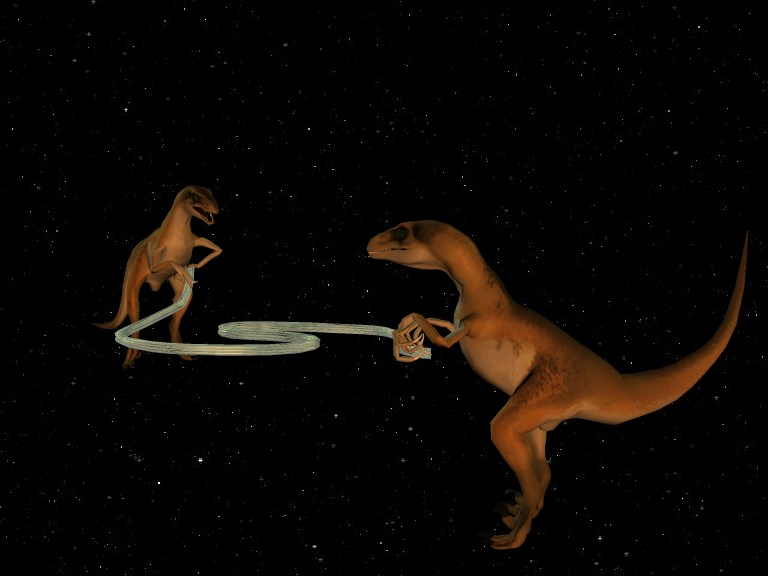The last theory explaining the structure of the stuff of Universe is the toe ( theory of Everything ). According to this theory, fundamental particle of universe are little strings, vibrating like music tools ropes. According to the vibrations, we can see: Neutrons, Protons etc...

What is important for us is... that theory allows sudden changes of the value of G!

What is a "quantum jump"?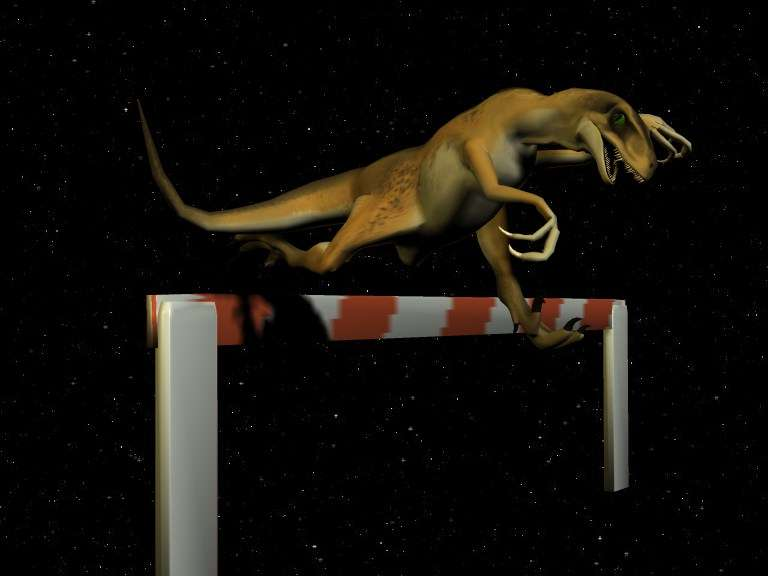In today's world you can observe the trajectory of a moving object. If you send a rockett from the Earth to the Moon, you'll see this rockett covering all the trajectory between Earth and Moon.
In the quantum physics world, rules are different.
An electron can "jump" between two levels of energy without going into "intermediate" levels. If the two levels of energy where Moon and Earth and the electron were a rockett,
you'd see the electron vanishing from the sights on Earth and appearing on the Moon.
It sounds like magic. But it's not magic, it's quantum physics.
We simply make here the hypothesis that G jumps like a common electron!